Calculator of open channel flow.APP Information

 Download Version 1.1 (2) Apk Size 1.28 MB App Developer Žiga Žibert Malware Check TRUSTED Install on Android 4.0.x and up App Package si.ziga.flowcalculator.apk MD5 916091bc48b74c761116682dc9898051 Rate 5 Website https://www.facebook.com/FlowCalculator### App Description

```Flow Calculator is calculator of open channel flow. App calculates Manning equation for different channel shapes (user can choose different parameters of equation to be calculated). App supports rectangular, trapezoidal, triangular and circular channel shape.

App also contains short theory behind Manning equation and table with values of all Manning coefficients. Manning equation is empirical equation estimating open channel flow:

v = 1/n*R^(2/3)*S^(1/2)

where:

v...cross section average velocity (m/s)
n...Manning coefficient
S...slope of water surface (m/m)

User can choose different parameters to be calculated for open channel flow:

RECTANGULAR CHANNEL
- velocity(v) & discharge(Q)
- channel slope(S) from velocity(v)
- channel slope(S) from discharge(Q)
- manning coefficient(n) from velocity(v)
- manning coefficient(n) from discharge(Q)
- water depth(h) from discharge(Q)

TRAPEZOIDAL CHANNEL
- velocity(v) & discharge(Q)
- channel slope(S) from velocity(v)
- channel slope(S) from discharge(Q)
- manning coefficient(n) from velocity(v)
- manning coefficient(n) from discharge(Q)
- water depth(h) from discharge(Q)
- bank slope(m) from discharge(Q)

TRIANGULAR CHANNEL
- velocity(v) & discharge(Q)
- channel slope(S) from velocity(v)
- channel slope(S) from discharge(Q)
- manning coefficient(n) from velocity(v)
- manning coefficient(n) from discharge(Q)
- water depth(h) from discharge(Q)
- bank slope(m) from discharge(Q)

CIRCULAR CHANNEL
- velocity(v) & discharge(Q)
- channel slope(S) from velocity(v)
- channel slope(S) from discharge(Q)
- manning coefficient(n) from velocity(v)
- manning coefficient(n) from discharge(Q)
- water depth(h) from discharge(Q)
```

### App ChangeLog

• - support for imperial units (US)
• - unit converter is added
• - user interface tweaks

### More Android Apps to Consider

1.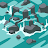JULIAN NAVAS MARTÍNEZ

Great free app, works just fine

2.Nelius Van Der Merwe

Excellent on hand calculator wuth accurate results

3.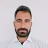Great app thank you very much

4.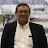Irwan Joe

Easy to calculate

5.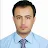Amir Baig

Well I am satisfied with this app

6.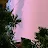Hasnan Marwazi

Good, but I have different result when using spreadsheet excell, especially for flow volume.

7.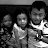Gatot Nugroho

Simple, easy to use, save my time. Thank you!

8.Charlie Oppliger

Helpful for quick calculations, has a nice interface and doesn't break

9.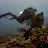Bimo Irianto

Sangat berguna dan bermanfaat untuk para praktisi

10.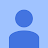Dennis Daniel

Sir can you Create Flow Rate Calculator?